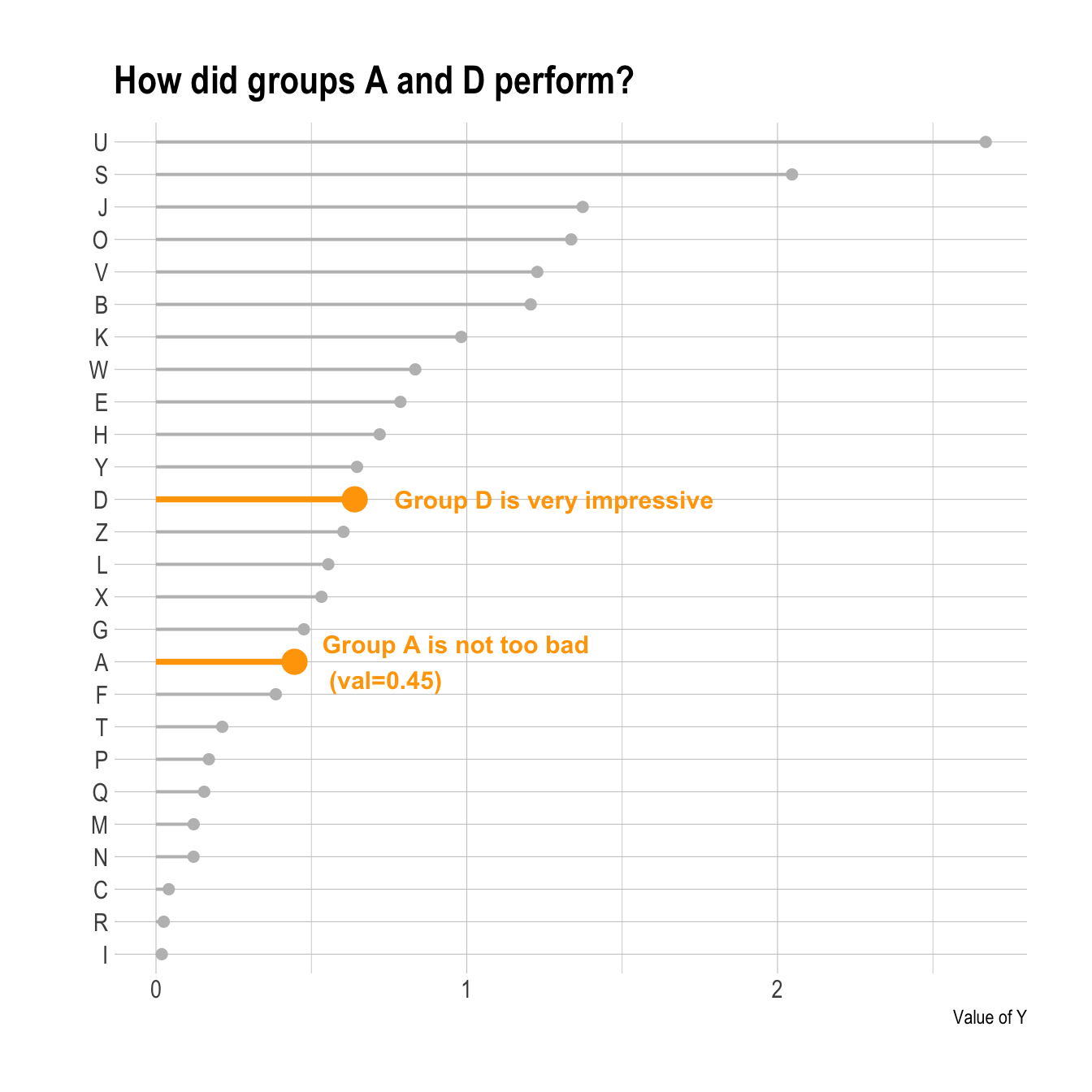# Highlight a group in a lollipop chart

This post describes how to build a lollipop chart with `R` and `ggplot2`. It shows how to highlight one or several groups of interest to convey your message more efficiently.

Annotation is key in data visualization: it allows the reader to focus on the main message you want to convey.

If one or a few groups specifically interest you, it is a good practice to highlight them on the plot. Your reader will understand quicker what the story behind the chart is.

To do so, you can use an `ifelse` statement to change `size`, `color`, `alpha` or any other aesthetics. Moreover, it is even more insightful to add text annotation directly on the chart.``````# Library
library(ggplot2)
library(dplyr)
library(hrbrthemes)

# Create data
set.seed(1000)
data <- data.frame(
x=LETTERS[1:26],
y=abs(rnorm(26))
)

# Reorder the data
data <- data %>%
arrange(y) %>%
mutate(x=factor(x,x))

# Plot
p <- ggplot(data, aes(x=x, y=y)) +
geom_segment(
aes(x=x, xend=x, y=0, yend=y),
color=ifelse(data\$x %in% c("A","D"), "orange", "grey"),
size=ifelse(data\$x %in% c("A","D"), 1.3, 0.7)
) +
geom_point(
color=ifelse(data\$x %in% c("A","D"), "orange", "grey"),
size=ifelse(data\$x %in% c("A","D"), 5, 2)
) +
theme_ipsum() +
coord_flip() +
theme(
legend.position="none"
) +
xlab("") +
ylab("Value of Y") +
ggtitle("How did groups A and D perform?")

p + annotate("text", x=grep("D", data\$x), y=data\$y[which(data\$x=="D")]*1.2,
label="Group D is very impressive",
color="orange", size=4 , angle=0, fontface="bold", hjust=0) +

annotate("text", x = grep("A", data\$x), y = data\$y[which(data\$x=="A")]*1.2,
label = paste("Group A is not too bad\n (val=",data\$y[which(data\$x=="A")] %>% round(2),")",sep="" ) ,
color="orange", size=4 , angle=0, fontface="bold", hjust=0) ``````

# What’s next

The lollipop chart is one of my favourite. There is so much to do with it and it is under-utilized in favor of barplot. Visit the dedicated section for more examples produced with `R`, or data-to-viz to learn about the available variations and caveats to avoid.

Related chart types

## Contact

This document is a work by Yan Holtz. Any feedback is highly encouraged. You can fill an issue on Github, drop me a message on Twitter, or send an email pasting yan.holtz.data with gmail.com.